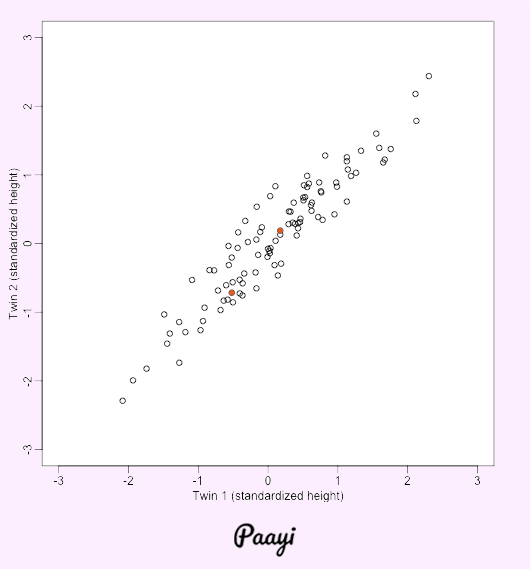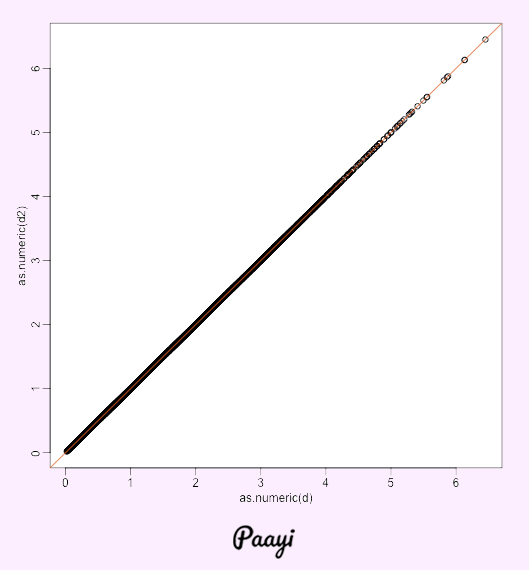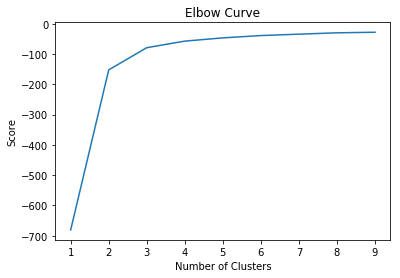## K-Means Clustering in Python

##### In this part of Learning Python we Cover K-Means Clustering In Python
Written by |14-May-2019 | 0 Comments | 567 Views

Dimensionality Reduction Motivation:

Visualization of data is one of the critical methods to understand the data. However, sometimes it is tough to visualize the data because the data is in nth dimension and all these vectors can be visualized on a screen. We can only visualize the up to 3d beyond this we are not able to visualize. For such we have to reduce the data.

We have appeared for visualizing global properties of the sections of lines, yet plots that uncover connections between segments or between lines are progressively entangled because of the high dimensionality of information.

Assume we have information with n measurements. It will be difficult to imagine that information, so we diminish the measurement from n to 2 measurements to picture the information

Reducing 2D to 1D:

We consider an example with twin heights. Here we simulate 100 two dimensional points that represent the number of standard deviations each is from the mean height. Each pair of points is a twins:​​​​​

 Figure 1

The data will look like this. However, by applying PCA( Principal Component Analysis ), the data will be converted into a straight line by minimizing the magnitude x. Moreover, the data will look like this.Figure 2

It is to be noted that PCA is not related to linear regression. In case linear regression y is predicted, and it minimizes the square distance between continuous values. Whereas in PCA no Y is predicted a line minimizes the magnitude so that the x can be represented as 1-dimensional data.

Reducing nD to 2D:

Similarly, we reduce the data nD to 2D suppose we have data containing the economic conditions of the country. If we plot that data, we will not be able to visualize the data or understand that data. So we reduce the data to 2D. Also, that visualization will be easy to understand.

We reduce the data from n dimension to k dimension by computing covariance matrix which is given by

sigma = 1/m sum( 1 to n ) ( x^i ) ( x^i )^T

Uses:

• Less Complexity of data
• Better Visualization
• Reduce Size

K-Means by Hand

DataSet = {2, 3, 4, 10, 11, 12, 20, 25, 30} perform K-means cluster when k=2

suppose we have data given above and k=2 means we have to make two clusters.

First, we initialize two centroid position randomly by taking:

m1=4, m2=12

Now calculate each index of the data and put the element in K1 if the distance is less from m1 and put into K2 if the distance is less than m2.

First Iteration:

K1 = {2, 3, 4}                         K2 = {10, 11, 12, 20, 25, 30}

Find Mean of K1 and K2

now m1 = 3 and m2 = 18

Second Iteration:

K1 = {2,3,4,10 }            K2 = {11,12,20,25,30}

Find Mean of K1 and K2

now m1 = 5 and m2 = 20

Third Iteration:

K1 = {2,3,4,10,11,12 }            K2 = {20,25,30}

Find Mean of K1 and K2

now m1 = 7 and m2 = 25

Fourth Iteration:

K1 = {2,3,4,10,11,12 }            K2 = {20,25,30}

Find Mean of K1 and K2

now m1 = 7 and m2 = 25

m1 and m2 are same as above so we stop our iteration here.

Moreover, we got two clusters that are K1 and K2.

Practical Implementation of K-Means:

Now let's dig into the code of K means clustering. We will take the same dataset that we have used before the iris dataset, but this time we only use the features and will not use the targets because as we know that in unsupervised learning the data is not labeled.

So start the code.

`from sklearn.datasets import load_iris`
`from sklearn.cross_validation import train_test_split`
`from sklearn.cluster import KMeans`
`import matplotlib.pyplot as plt`
`from sklearn import metrics`

First, we import all of the modules.

`data = load_iris()`
`X = data.data`

Then we load the data and only took the data not the names of the targets.

`num = range(1, 10)`
`kmeans = [KMeans(n_clusters=i) for i in num]`
`score = [kmeans[i].fit(X).score(X) for i in range(len(kmeans))]`
`plt.plot(num,score)`
`plt.xlabel('Number of Clusters')`
`plt.ylabel('Score')`
`plt.title('Elbow Curve')`
`plt.show()`

As we know that in the iris data set we have three classes, but here we don't know how many classes are there and if we do not know how will we allocate the clusters. For this we have to evaluate how many clusters should we give to make a cluster. Elbow method will show us how much cluster should we define. The above code is for having the number of clusters by the elbow method the graph is as follows:Figure 3

In the above figure, we see a sharp curve at 3 so we can deduce from it that there are 3 clusters means three classes.

And now to train the model we use the following method:

`kmeans=KMeans(n_clusters=3)`
`model=kmeans.fit(X)`
`model.labels_`

 array([0, 0, 0, 0, 0, 0, 0, 0, 0, 0, 0, 0, 0, 0, 0, 0, 0, 0, 0, 0, 0, 0,        0, 0, 0, 0, 0, 0, 0, 0, 0, 0, 0, 0, 0, 0, 0, 0, 0, 0, 0, 0, 0, 0,        0, 0, 0, 0, 0, 0, 1, 1, 2, 1, 1, 1, 1, 1, 1, 1, 1, 1, 1, 1, 1, 1,        1, 1, 1, 1, 1, 1, 1, 1, 1, 1, 1, 2, 1, 1, 1, 1, 1, 1, 1, 1, 1, 1,        1, 1, 1, 1, 1, 1, 1, 1, 1, 1, 1, 1, 2, 1, 2, 2, 2, 2, 1, 2, 2, 2,        2, 2, 2, 1, 1, 2, 2, 2, 2, 1, 2, 1, 2, 1, 2, 2, 1, 1, 2, 2, 2, 2,        2, 1, 2, 2, 2, 2, 1, 2, 2, 2, 1, 2, 2, 2, 1, 2, 2, 1], dtype=int32)

In this way we can use k means we can then name the clusters. We can see that the labels are quite good. The 0 which is setosa in standard cases is identified. There are some miscalculation between 1 and 2, but this is all right in the case of clustering.

So in this document, we have seen the practical implementation of K-Means, and in the next section we will see the hierarchical clustering.